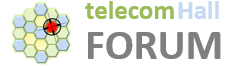# How can we measure the noise on Uplink in 5G?

Hello Experts.

How can we measure the noise on Uplink in 5G?

Is it same like LTE, we have KPI Avg UL SINR PUSCH/PUCCH.

And there are counters for this per each PRB.

For Nokia refer MEAN_UL_RIP_PER_PRB counters on PRB level else mean RSSI on cell level.

So how is it calculated?
I mean the value of Mean_UL_RIP_PER_PRB, how is it calculated?

The average I+N for each PRB is the total of I+N from the available layers for each PRB divided by the number of available layers for each PRB.

This counter is updated within the measurement period with the arithmetical average value of samples, each sample presenting the average RIP in the uplink subframe over 100 ms for PRB.